# Geometry 11 7 Ratio of Areas Comparing Areas

• Slides: 10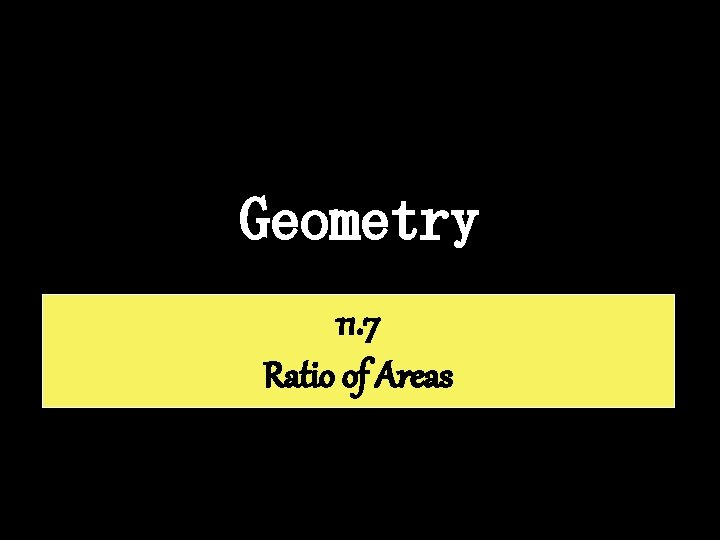Geometry 11. 7 Ratio of AreasComparing Areas of Triangles If two triangles have equal heights, then the ratio of their areas equals the ratio of their bases. A = ½(10)(12) = 60 12 10 Ratio of bases: 10 = 1 20 2 A = ½(20)(12) = 120 12 20 Ratio of areas: 60 = 1 120 2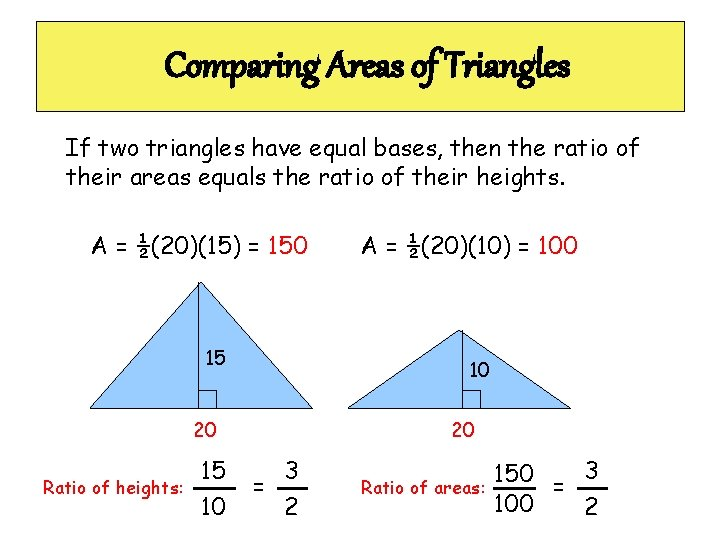Comparing Areas of Triangles If two triangles have equal bases, then the ratio of their areas equals the ratio of their heights. A = ½(20)(15) = 150 15 10 20 Ratio of heights: 15 10 A = ½(20)(10) = 100 20 3 = 2 Ratio of areas: 150 = 3 100 2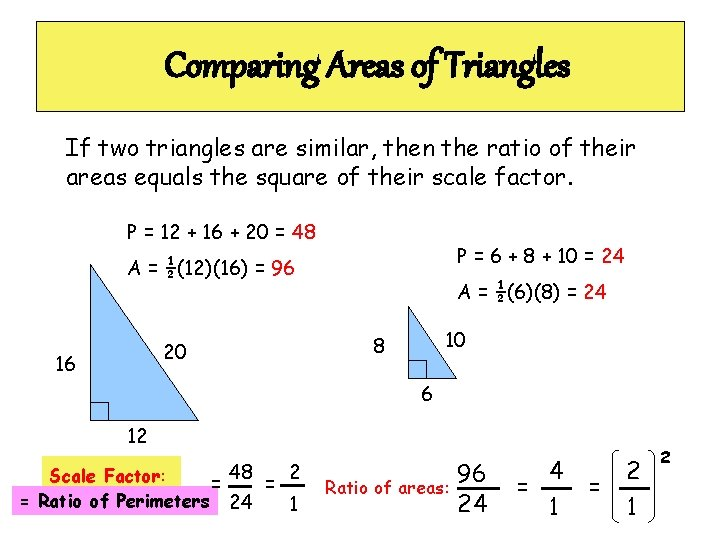Comparing Areas of Triangles If two triangles are similar, then the ratio of their areas equals the square of their scale factor. P = 12 + 16 + 20 = 48 P = 6 + 8 + 10 = 24 A = ½(12)(16) = 96 20 16 A = ½(6)(8) = 24 10 8 6 12 48 2 Scale Factor: = = = Ratio of Perimeters 24 1 Ratio of areas: 96 24 4 = 1 = 2 1 2What to ask yourself!!! 1) Do the triangles have the same height? – If yes, the ratio of the areas is the ratio of the bases. 2) Do the triangles have the same base? – If yes, the ratio of the areas is the ratio of the heights. 3) Are the figures similar? – If yes, the ratio of the areas is the square of the scale factor.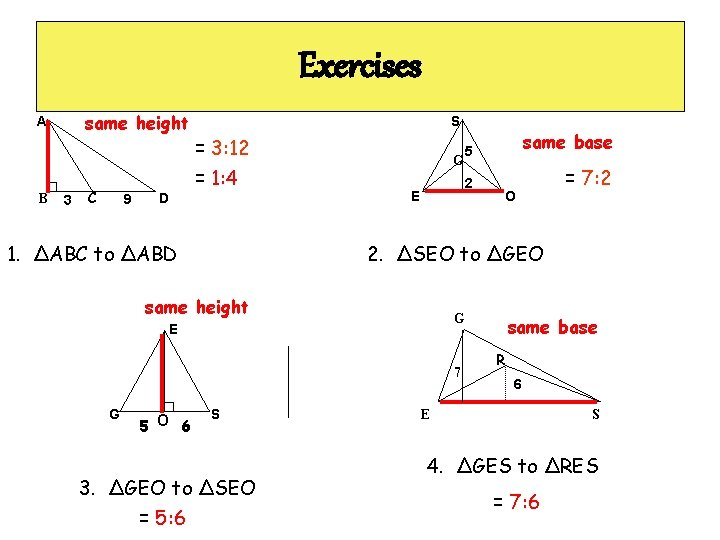Exercises same height A B 3 C 9 D S = 3: 12 = 1: 4 1. ∆ABC to ∆ABD E 2 = 7: 2 O 2. ∆SEO to ∆GEO G E 7 5 O 6 same base G same height G 5 S 3. ∆GEO to ∆SEO = 5: 6 same base R 6 E S 4. ∆GES to ∆RES = 7: 6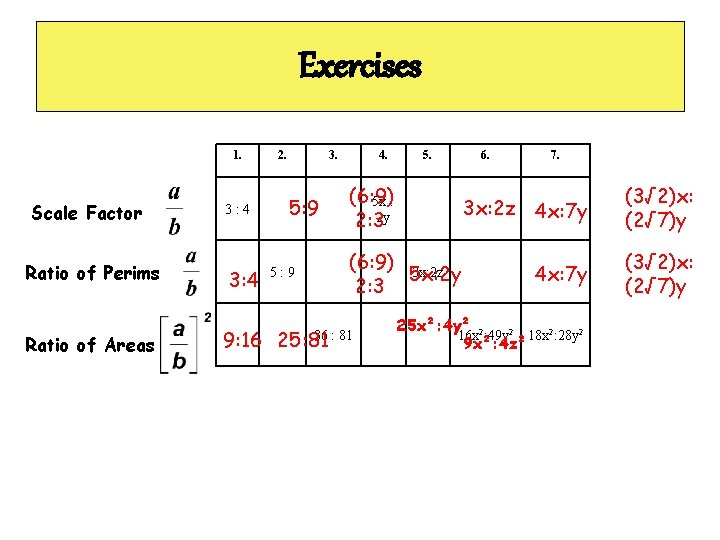Exercises 1. Scale Factor Ratio of Perims Ratio of Areas 3: 4 9: 16 2. 3. 5: 9 4. 5. (6: 9) 5 x : 2: 32 y (6: 9) 3 x: 2 z 5 x: 2 y 2: 3 36 : 81 25: 81 6. 7. 3 x: 2 z 4 x: 7 y (3√ 2)x: (2√ 7)y 25 x²: 4 y² 2 16 x : 49 y 2 18 x 2: 28 y 2 9 x²: 4 z²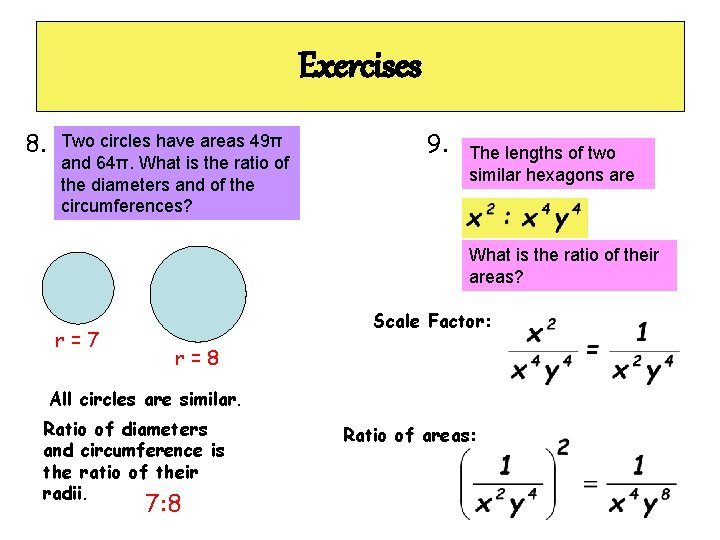Exercises 8. Two circles have areas 49π and 64π. What is the ratio of the diameters and of the circumferences? 9. The lengths of two similar hexagons are What is the ratio of their areas? r=7 Scale Factor: r=8 All circles are similar. Ratio of diameters and circumference is the ratio of their radii. 7: 8 Ratio of areas:One from the HW • P. 458 CE #15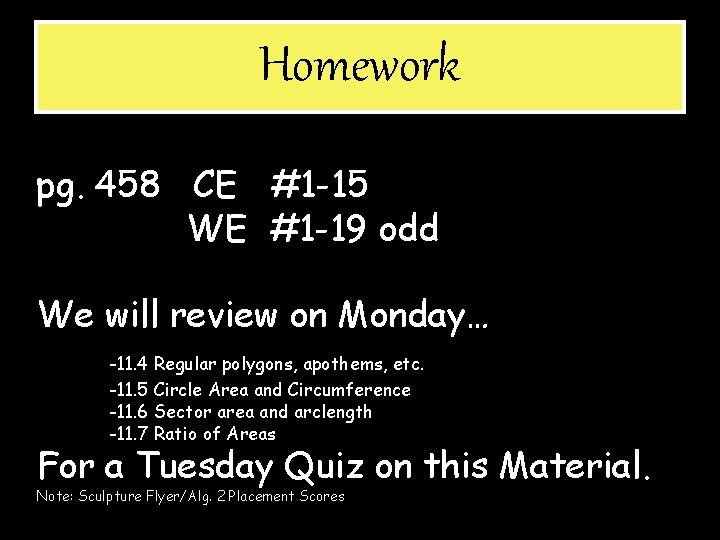Homework pg. 458 CE #1 -15 WE #1 -19 odd We will review on Monday… -11. 4 Regular polygons, apothems, etc. -11. 5 Circle Area and Circumference -11. 6 Sector area and arclength -11. 7 Ratio of Areas For a Tuesday Quiz on this Material. Note: Sculpture Flyer/Alg. 2 Placement Scores